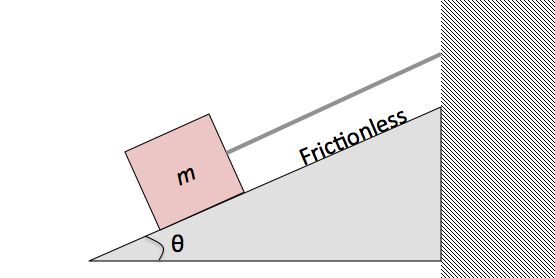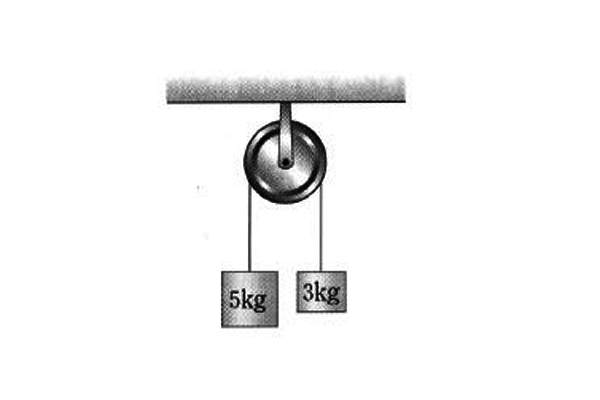Classical Mechanics

# Newton's Second LawIn the figure above, a block on a frictionless slope is hanging on a cord. The mass of the block is $$m= 4.0 \text{ kg},$$ and the slope makes a $$\theta=30 ^\circ$$ angle with the horizontal. If the cord is cut, what is the magnitude of the acceleration of the block?

The gravitational acceleration is $$g= 10 \text{ m/s}^2.$$A $$900 \text{-kg}$$ rocket sled is to be accelerated from rest to $$1700 \text{ km/h}$$ at a constant rate in $$1.8$$ seconds in outer space. What is the approximate magnitude of the required net force?

If a $$5.00 \text{ kg}$$ point mass has an acceleration of $$10.00 \text{ m/s}^2$$ at $$30 ^{\circ}$$ from the positive direction of the $$x$$-axis, what is the net force in unit-vector notation?

In the movie Spiderman 2, there's a famous minute long scene where Spiderman stops a train. If the initial speed of a five-car, out of control subway train was around the top speed of the NYC subway (27 m/s), and Spiderman stopped the train in exactly one minute, then what is the magnitude of the force in Newtons that Spiderman exerted on the train as he stopped it?

Details and assumptions

• A standard New York City subway car full of terrified passengers has a mass of roughly 40,000 kg.
• You may assume the acceleration was constant and any other forces are negligible.In the above diagram, two objects with their respective masses $$5$$ kg and $$3$$ kg are hung on a pulley with no friction. Find the magnitude of acceleration $$(\text{in m/s}^2 )$$ of the left object with mass $$5$$ kg.

Gravitational acceleration is $$g = 10$$ m/s$$^{2}$$.

×

Problem Loading...

Note Loading...

Set Loading...